# NCERT Solutions for Class 8 Maths Chapter 12 Exponents and Powers

NCERT Solutions for Class 8 Maths Chapter 12 Exponents and Powers Exercise 12.1

Ex 12.1 Class 8 Maths Question 1.
Evaluate:
(i) 3-2
(ii) (-4)-2
(iii) ($$\frac { 1 }{ 2 }$$)-5
Solution: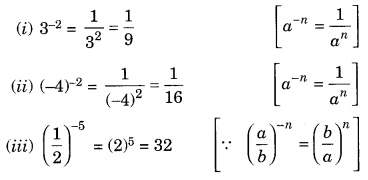Ex 12.1 Class 8 Maths Question 2.
Simplify and express the result in power notation with a positive exponent.Solution:Ex 12.1 Class 8 Maths Question 3.
Find the value of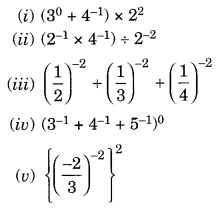Solution: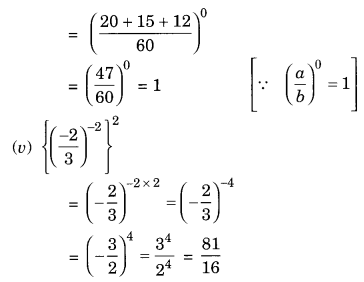Ex 12.1 Class 8 Maths Question 4.Solution: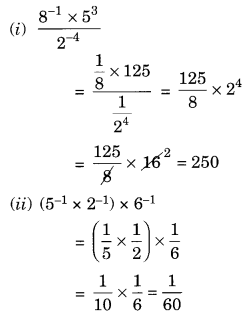Ex 12.1 Class 8 Maths Question 5.
Find the value of m for which 5m ÷ 5-3 = 55.
Solution:
5m ÷ 5-3 = 55
⇒ 5m-(-3) = 55 [∵ am ÷ an = am-n]
⇒ 5m+3 = 55
Comparing the powers of equal bases, we have
m + 3 = 5
m = 5 – 3 = 2, i.e., m = 2

Ex 12.1 Class 8 Maths Question 6.
Evaluate: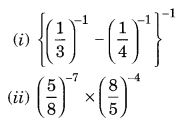Solution: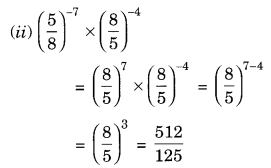Ex 12.1 Class 8 Maths Question 7.
Simplify:Solution: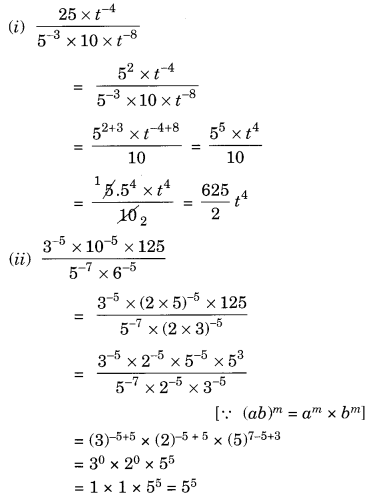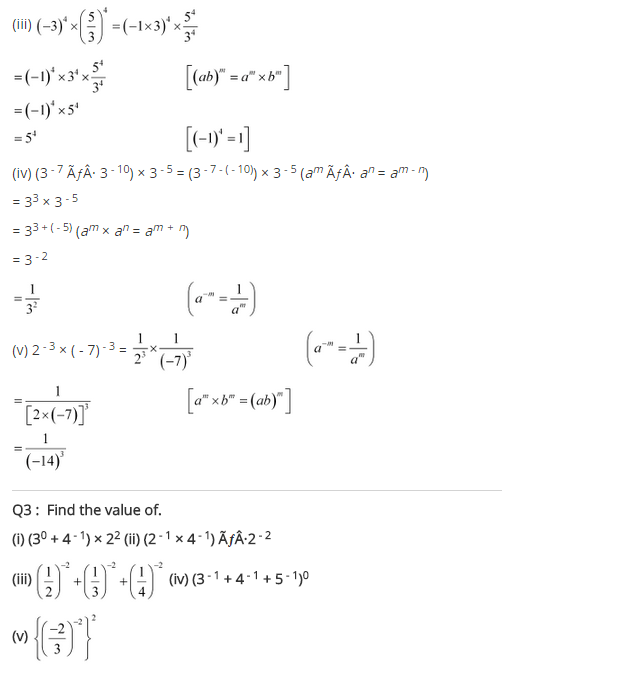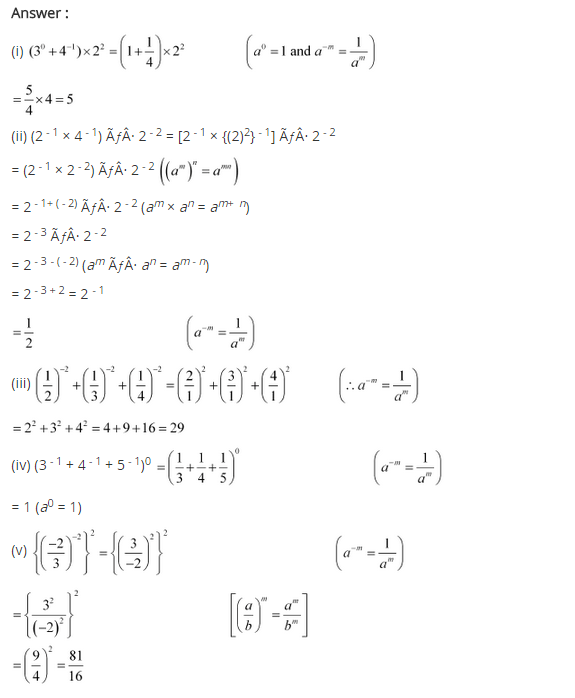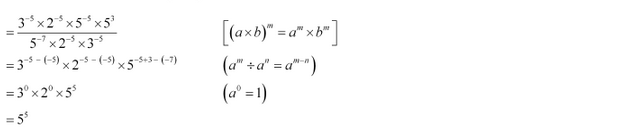+